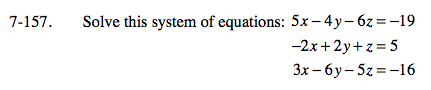Home > A2C > Chapter 7 > Lesson 7.3.1 > Problem7-157

7-157.

Solve this system of equations: Homework Help ✎ 5x − 4y − 6z = −19
−2x + 2y + z = 5
3x − 6y − 5z = −16Use elimination to eliminate y from equations 1 and 2.

Use elimination to eliminate y from equations 2 and 3.

Use your two new equations to solve for x and z. Don't forget to solve for y as well.